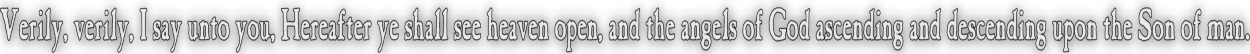None:
Polyps:
Strongs:CreationismI Didn't Put My Name To That! When we looked at the repeated application of the dialectic we noted that opposing the process and shifting one's fixed point to be in opposition to the process itself left the antagonist in a position where the process itself leads to the individual having to reject their own position to reject the process. The only way to oppose the process is not to shift ones view at any time. If we start with say, {e,f,g} = ¬ {d,e,f} i.e. d = ¬g {e,f,g} => {d,e,g} <= {d,e,f} with oil "f" and wine "e" The opposing view is the remaining {d,f,g} Then the result leads to {d,e,g} = ¬ {d,f,g} and say the further balance of {d,f,g} => {d,e,f} <= {d,e,g} and the objection {d,e,f} = ¬{e,f,g}, our original balance. However if one takes part in the process the attempt is made to continually propose syntheses to make the fixed position compromise. Thus, starting with {e,f,g} = ¬ {d,e,f} {e,f,g} => {d,e,g} <= {d,e,f} leads on rejection of the synthesis to {d,e,g} = ¬ {d,e,f} and then because we wish ¬{e,f,g} {d,e,g} => {d,f,g} <= {d,e,f} leads on rejection to {d,f,g} = ¬ {d,e,f} and then because we wish ¬{d,e,g} {d,f,g} => {e,f,g} <= {d,e,f} leading to the original {e,f,g} = ¬ {d,e,f} taking a look at the oil and wine, sending oil to {}. e wine and f oil d wine and e oil f wine and d oil There is the appearance that g is remaining as oil, with either d, e or f as wine. The result shows that {d,f,g} => {d,e,f} <= {d,e,g} and {d,f,g} => {d,e,f} <= {e,f,g} or {e,f,g} => {d,e,f} <= {d,e,g} remain ; and then say, having rejected both {e,f,g} and {d,e,g} we could imply the individual reject {d,e,f} also, their original position. In remaining fixed the individual has opposed the sending of any of their singletons to {}. If the individual would permit g=>{} then they set themselves up to be the synthesis of any combination of the rejected syntheses. Similar and perhaps to follow is the result that {e,f,g} => {d,e,g} <= {d,e,f} excludes {d,f,g} {d,f,g} => {d,e,g} <= {d,e,f} excludes {e,f,g} {d,f,g} => {d,e,g} <= {e,f,g} excludes {d,e,f} and the excluded triples in synthesis exclude {d,e,g} rejecting the balances would lead to any balance of the excluded views, to reject this extra balance for its exclusion would give {d,e,g} in this case, which is the result of the initial three balances resulting in {d,e,g}. Now which is preferable? Opposing the argument and where it goes, or to oppose the process? In both cases the result is that engaging in the process is not valuable, In the first instance the discourse will go around in crcles indefinitely, and in the second case the dialectic may be shown to have sent every element in {d,e,g} to {} sequentially. In both cases it may be pointed out that the system has shown self-inconsistency: That in the first case the method is unable to determine the truth or falsity of {d,e,f} and the second case must assume that every argument has accumulated the sending of every element to {}. It is irrelevant in the first case to assume that g=>{} may determine the efficacy "of the method" in support of {d,e,f} and it is irrelevant in the second case to assume that {d,e,g} is therefore correct by eliminating the methods own inconsistency after the elements d, e and f have all been sent to {} arbitrarily. It makes sense to continue to exclude {d,e,f}={} and state the method is inconsistent! Truth then is not obtained by the method, but by whom may press the hardest or whom should be excluded for appearing to be "not a team player". Likewise in the octal we have to state that one element only is the identity element of unity so if this is "f" say then we have the balances of; {e,f,g} => {d,e,g} <= {d,e,f} excludes {d,f,g} {d,f,g} => {d,e,g} <= {d,e,f} excludes {e,f,g} {d,f,g} => {d,e,g} <= {e,f,g} excludes {d,e,f} and the excluded triples in synthesis exclude {d,e,g} And these are the true result of not shifting the fixed position. However the former case is that; {e,f,g} => {d,e,g} <= {d,e,f} leads to {d,e,g} = ¬ {d,e,f} {d,e,g} => {d,f,g} <= {d,e,f} leads to {d,f,g} = ¬ {d,e,f} {d,f,g} => {e,f,g} <= {d,e,f} leads to {e,f,g} = ¬ {d,e,f} The case here is not in staying fixed; rather the fixed position has shifted from "f" to "e" to "d". Whereas the antithesis has not shifted, it has engaged in the method; In both cases holding one's views fixed is less preferable than not to engage at all. In both cases there is a cyclic arrangement of thesis/antithesis or of the synthesis becoming a new thesis. Neither of these cyclic arrangements resolve the truth of the argument - but if the absolute truth is indeed the correct and fixed position, there is no strength in the dialectic method against it. The method simply cycles arbitrarily and reaches nothing new. It pays to pay attention then - for if an argument is contradicted then when the method later cycles and repeats the fact it may be correctly stated that the opposition is inconsistent. One could state "four strikes, you're out!" Return To Section Start Return To Previous Page'